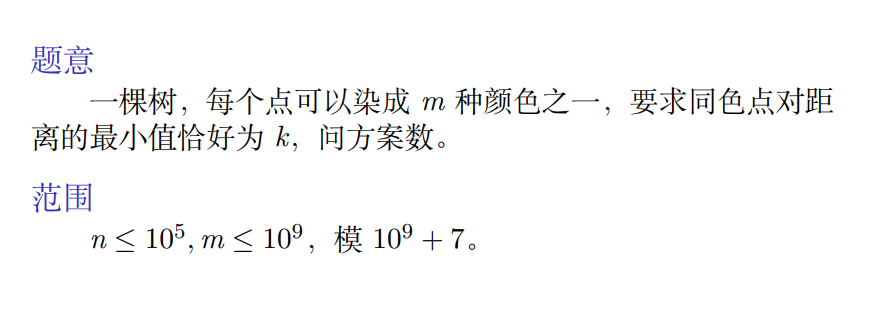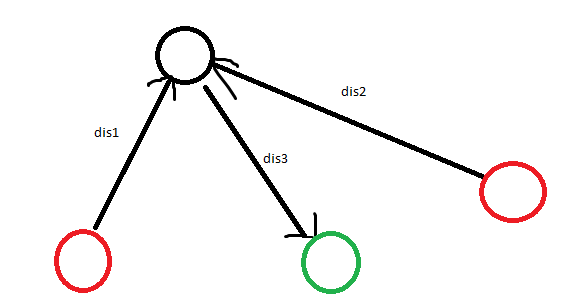10.29 写题

## CF388D

• $k = 0$

这一位可以随便填或者不填。如果这一位填了 $1$ ，那么之前位的这一位都不能有值。转移系数是 $1$ 。如果这一位不填 $1$ ，之前可以任意填，转移系数 $2^j$。

• $k = 1$

如果填入这一位，则仍然是卡着上界的。转移系数是 $1$ 。

如果不填，则如果之前这一位上有偶数个 $1$ ，那么就不再卡着上界了。转移系数是 $\binom n 0 + \binom n 2 + \dots = 2^{j-1}$ ，因为对于所有选择偶数个数的方案，我们只要把第一个位置给反转一下，就一定可以得到一个选择奇数个数的方案，并且明显方案一定两两对应。

如果之前这一位上有奇数个 $1$ ，那么仍然卡着上界，系数是 $2^{j-1}$ 。

## 染色